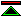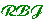The Formalisation of Mathematics

 During the period from about 1821 to 1908, which begins with the publication of Cauchy's Cours d'analyse algebraic and concludes with the publication in 1908 of Russell's Mathematical Logic as Based on The Theory of Types and Zermelo's first order axiomatisation of set theory, mathematicians restored and surpassed the standards of rigour which had been established during the period of classical greek mathematics but neglected during the mathematisation of science.

 The Rigourisation of Analysis The use of infinitesimals was one of the more conspicuous practices in the development of analysis for which no justification or rationale could be offered. The rigourisation of analysis begins with the elimination of infinitesimals in favour of arguments using limits. Frege's Logic For millenia mathematics had been a science based on deductive logic. But no account of logic had ever been produced which was adeqate for the purposes of mathematics. By abandoning Aristotle's subject-predicate analysis of the form of sentences, Frege was able to devise a logic which would prove sufficient for the formalisation of mathematics. Grundgesetze and Principia Mathematica Frege's logic was intended to provide a foundation for mathematics, and Frege showed how this could be done in his Grundgesetze der Arithmetic. This however proved to be based on an inconsistent logical system. Russell, taking the paradoxes into account, devised his Theory of Types and demonstrated with Whitehead in Principia Mathematica how this could be used to formalise mathematics. Formal mathematics was shown to be rather hard work for human beings, and did not catch on. The Theory of Real Numbers The rigourisation of analysis preceded and showed the need for a better account of the real number system, since the necessary proofs of results in analysis depended on properties of real numbers which were not themselves rigorously established. Cantorian Set Theory Both the theory of real numbers and the idea of a function depended upon an informal notion of set. Cantor turned the very simple idea of a set into a rich theory which was to become the foundation of modern mathematics. Zermelo's Axiomatic Set Theory After the paradoxes became conspicuous in set theory it became essential to have a consistent axiomatisation of the theory if it was to be used. Zermelo's axiomatisation was the first, and with some subsequent improvements became the de-facto standard foundation for mathematics. Though Zermelo's account was informal it is a small step from there for a fully formal account of mathematics as based on first order set theory.

Formality and Rigour in the 20th Century©created 1996/8/11 modified 1996/10/5